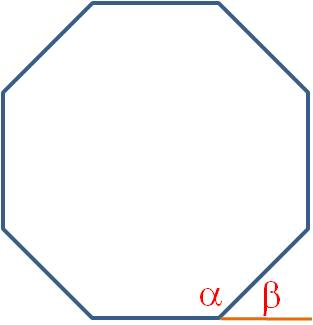## finding internal or external angles of regular polygons

How do you find the measure of an internal angle or an external angle on a regular polygon? It’s quite easy, if you memorize two formulas. All you have to do is count the number of sides on the polygon and plug it into the formula.

For internal angles, use the formula$\alpha = \dfrac{180(n-2)}{n}$

For external angles, the formula is even easier:$\beta = \dfrac{360}{n}$

For example, here is a regular octagon, so n = 8. The internal angle is labeled α and the external angle is β.$\alpha = \dfrac{180(8-2)}{8}=135 \textdegree$$\beta = \dfrac{360}{8} = 45 \textdegree$

And here’s a trick to save you some time: You can see in the figure that the internal and external angles are always supplementary. (This is true in any polygon, even if it isn’t regular.) So if you need to find the internal angle, you can find the external angle instead with the simpler formula. Then the supplement to that angle is the internal angle!

Geometry
Blue Taste Theme created by Jabox